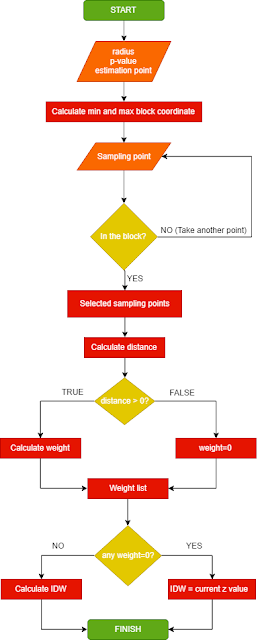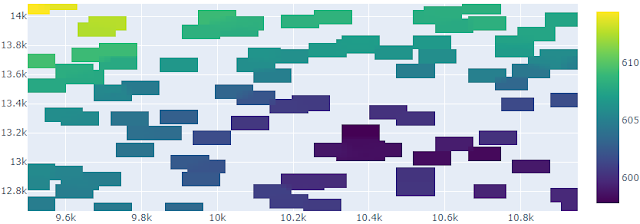# Creating IDW Interpolation from Scratch in Python

In another post we had discussed about Inverse Distance Weight (IDW) spatial interpolation which covered some topics such as IDW interpolation method, implementation concept of IDW interpolation in GIS software and how to do IDW interpolation using QGIS. In this post we will make our own IDW interpolation function from scratch using Python. After reading this tutorial you will be able to identify two approaches in selecting sample point to be used in IDW computation, creating python function and implementing the function. For this tutorial I used Jupyter lab 1.0 with Python 3.7.4, Numpy  1.16.4 and Plotly 4.1.0.

### IDW Algorithm

To estimate an unknown point, it's required some sampling points around. The question is how to select the neighborhood sampling points? There are two approaches that can be implemented for selecting the neighborhood point, the first one is based on a specified radius from an unknown point and the second one is selecting a number or a minimum number of points around the unknown point. In this tutorial we will discuss both of them.

#### IDW Interpolation Algorithm Based on Block Radius Sampling Point

The flow chart in figure 1(click to enlarge) shows step by step approach to determine an estimated value at an unknown point. For this algorithm the radius (r), p-value and estimation/unknown coordinate point are defined first. Then the block area will be defined with minimum and maximum coordinate. The center of the block will be the unknown point coordinate (xz,yz). The maximum and minimum of the block coordinate will be calculated as follow:

```x_min=xz-r
x_max=xz+r
y_min=yz-r
y_max=yz+r
```Figure 1. IDW Flowchart 1
After getting the minimum and maximum coordinate of a respective block, then each sampling point (xs[i],ys[i]) will be tested if it lies between the max-min block coordinate with the following condition.
```((xs[i]>=x_min and xs[i]<=x_max) and (ys[i]>=y_min and ys[i]<=y_max))=True
```

If the condition returns True then the evaluated sampling point will be grouped and added in a selected point list.

Next step the algorithm will calculate the distance of each selected sampling point to the estimated point (center of the block).  Each distance will be checked if it is larger than 0. If  True/Yes, then the weight of the selected point will be calculated. Conversely, the weight will be 0. All the weight result will be stored in a list.

The weight list then will be checked if there is a 0 weight value exist. If the condition return True/Yes, then the estimated value will be the value on selected sampling point. Otherwise the algorithm will calculate estimated value by using IDW formula.

The code below is implementation of IDW algorithm with radius block approach.

 ``` 1 2 3 4 5 6 7 8 9 10 11 12 13 14 15 16 17 18 19 20 21 22 23 24 25 26 27 28 29 30 31 32 33 34 35``` ```def idw_rblock(xz,yz,r,p): x_block=[] y_block=[] z_block=[] xr_min=xz-r xr_max=xz+r yr_min=yz-r yr_max=yz+r for i in range(len(x)): # condition to test if a point is within the block if ((x[i]>=xr_min and x[i]<=xr_max) and (y[i]>=yr_min and y[i]<=yr_max)): x_block.append(x[i]) y_block.append(y[i]) z_block.append(z[i]) #calculate weight based on distance and p value w_list=[] for j in range(len(x_block)): d=distance(xz,yz,x_block[j],y_block[j]) #distance function is created outside this function if d>0: w=1/(d**p) w_list.append(w) z0=0 else: w_list.append(0) #if meet this condition, it means d<=0, weight is set to 0 #check if there is 0 in weight list w_check=0 in w_list if w_check==True: idx=w_list.index(0) # find index for weight=0 z_idw=z_block[idx] # set the value to the current sample value else: wt=np.transpose(w_list) z_idw=np.dot(z_block,wt)/sum(w_list) # idw calculation using dot product return z_idw ```

#### IDW Interpolation based on Minimum Number of Sampling PointFigure 2. Flowchart 2
The second algorithm is mainly the same with the first one. It will select sampling point around an estimation point based on a block radius. But this algorithm will start with an initial radius, then the radius will increase "x" unit at each iteration in a loop until the number of selected sampling point is equal or more than a defined number point. So the input variables for this algorithm are: initial radius, p-value, minimum number of point sampling and an a point to be estimated.

In figure 2(click to enlarge), can be seen the flowchart for the second algorithm. It can be observed from the figure that there are double loop exist in selecting sampling point stage. The first loop is to check if a sampling point is in a block, and the second loop is to check if the selected sampling point is equal or more than the defined number of point. If it returns True than the process will continue to calculate distance, weights, and determine IDW estimation value.

The code implementation for the second algorithm can be seen in the following code.

Is there any output difference between the two algorithm? We will see soon in the last part of this post.

 ``` 1 2 3 4 5 6 7 8 9 10 11 12 13 14 15 16 17 18 19 20 21 22 23 24 25 26 27 28 29 30 31 32 33 34 35 36 37 38 39 40``` ```def idw_npoint(xz,yz,n_point,p): r=10 #block radius iteration distance nf=0 while nf<=n_point: #will stop when np reaching at least n_point x_block=[] y_block=[] z_block=[] r +=10 # add 10 unit each iteration xr_min=xz-r xr_max=xz+r yr_min=yz-r yr_max=yz+r for i in range(len(x)): # condition to test if a point is within the block if ((x[i]>=xr_min and x[i]<=xr_max) and (y[i]>=yr_min and y[i]<=yr_max)): x_block.append(x[i]) y_block.append(y[i]) z_block.append(z[i]) nf=len(x_block) #calculate number of point in the block #calculate weight based on distance and p value w_list=[] for j in range(len(x_block)): d=distance(xz,yz,x_block[j],y_block[j]) if d>0: w=1/(d**p) w_list.append(w) z0=0 else: w_list.append(0) #if meet this condition, it means d<=0, weight is set to 0 #check if there is 0 in weight list w_check=0 in w_list if w_check==True: idx=w_list.index(0) # find index for weight=0 z_idw=z_block[idx] # set the value to the current sample value else: wt=np.transpose(w_list) z_idw=np.dot(z_block,wt)/sum(w_list) # idw calculation using dot product return z_idw ```

### IDW Algorithm Implementation in Python

Now it's time to implement those algorithms above. One example of the IDW function algorithm implementation can be found in a post about creating 3D terrain in Python. In the post I used the second approach which is estimated the height of interpolation point. In the post, 3D surface plot with Plotly library was used to construct 3D visualization of a terrain. But of course it can be switched to 2D using Plotly Heatmap or matplotlib pcolormesh.

 ```1 2 3 4``` ```#PLOTTING WITH PLOTLY fig=go.Figure() fig.add_trace(go.Heatmap(z=z,x=x,y=y,colorscale='Viridis')) fig.show() ```

Figure 3 and 4 shows the result of IDW interpolation using Plotly Heatmap. Figure 3 used the first algorithm with radius 50 m and p value 1.5. The other one used 5 minimum sampling point with the same p value. What happened with the result in figure 3? Why is it different with the other one, although they used exactly the same interpolation points?

It happened because for some points to be estimated, no sampling point can be found within radius 50 m. So we have to increase the radius to prevent this. On the other hand, it won't happen in the second approach, because the algorithm will do looping until it find a number of minimum sampling point for IDW interpolation.Figure 3. IDW interpolation result with 50 m radiusFigure 4. IDW interpolation result with minimum 5 samping point
That's all tutorial about creating IDW interpolation from scratch with Python. In this tutorial we had discussed two approaches in estimating an unknown value, created both interpolation function and also discussed the implementation of each algorithm. Thanks for reading and please share it if you think other people will get benefit from this article.